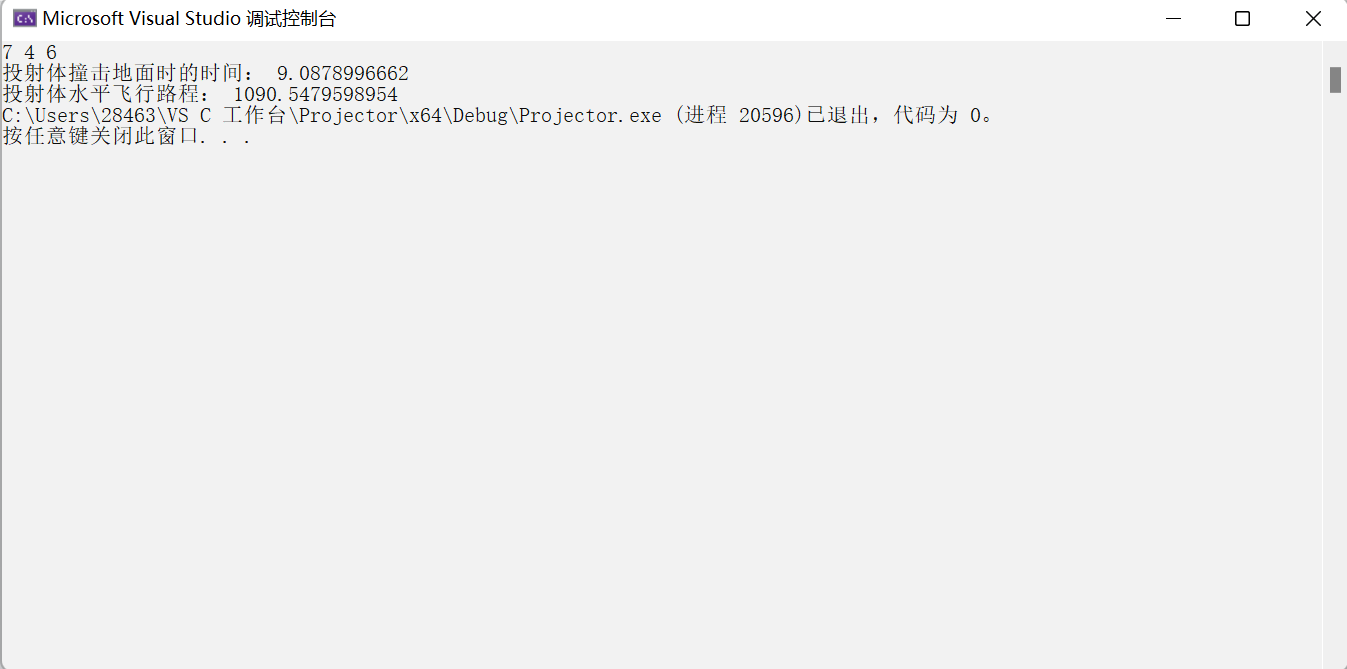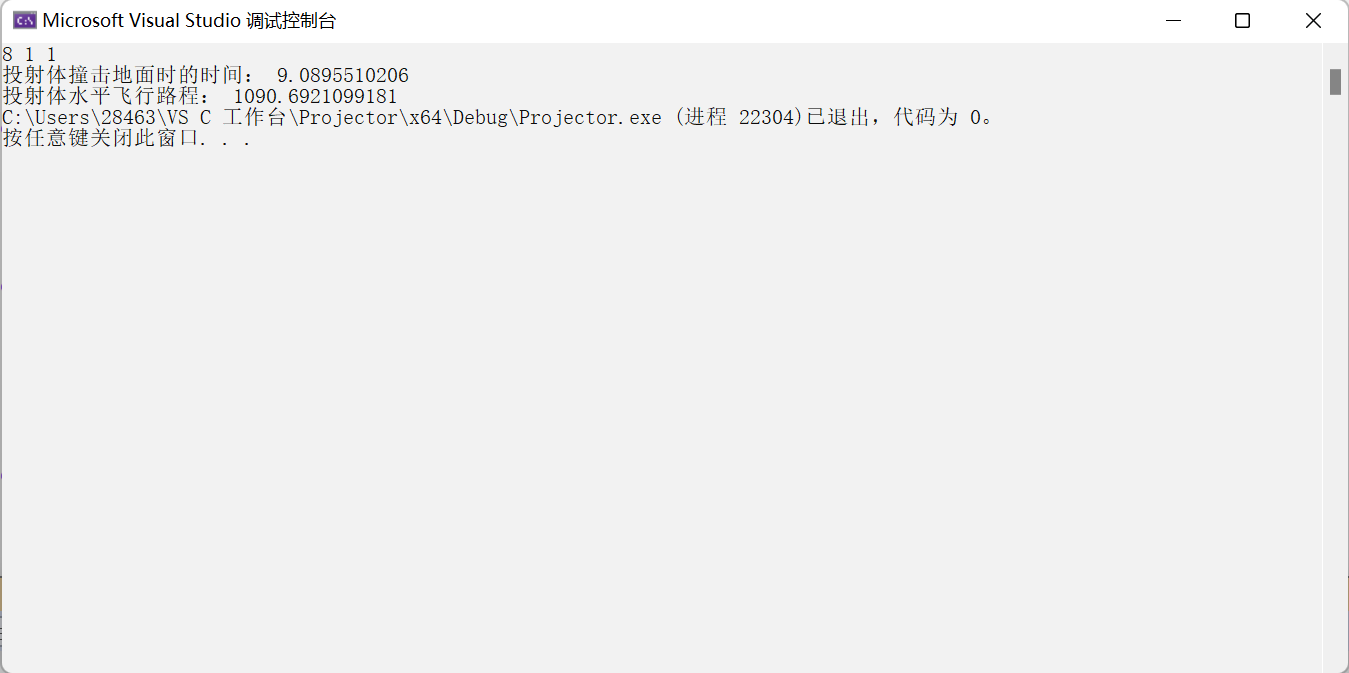> 文档中心 > 【数值计算】期末综合大作业

# 【数值计算】期末综合大作业🐲 1.提出问题

🦄1.1具体问题描述

🦄 1.2解决方案

🐲 2.分析问题

🦄 2.1基本思想

🦄 2.2基本原理

🐲 3.程序运行

🦄 3.1运行环境

🦄 3.2 程序代码

🦄 3.3 运行过程

🦄 3.4 运行结果

🐲 4.联系实际

【描述问题】

【输入形式】

【输出形式】

1. 分析问题
2. 采用的计算方法
3. 用编程来实现
4. 进行结果分析

# 🐲 2.分析问题

1. 目标函数最优化求解。
2. 进行方程的求解。

## 🦄 2.2基本原理

1. 前后两次迭代的差小于某一设定的精度
2. 与渐进误差常数有关
3. 与所给的函数的函数值精度有关

1. 先写主函数main() ，在函数体内部声明变量、精度、还有需要实现的函数功能，最后就是输出打印。
2. 用两个函数g()和h()对两个方程g(t)和h(t)进行实现，其中h()就是实现投射体水平飞行路程的函数
3. 再用函数g1()表示函数g(t)的求导方程
4. 计算投射体水平飞行路程，用函数newton()来实现，这个也是牛顿迭代法的核心

# 🐲 3.程序运行

## 🦄 3.2 程序代码

``#define _CRT_SECURE_NO_WARNINGS 1#include#include#includedouble e = 2.718281828;using namespace std;double g(double t){return 9600 * (1 - pow(e, (-t / 15.0))) - 480 * t;//y=g(t)=9600*(1-e^(-t/15.0)) - 480*t}double h(double t){return 2400 * (1 - pow(e, (-t / 15.0)));//x=h(t)=2400*(1-e^(-t/15.0))。}double g1(double t){return 640 * pow(e, (-t / 15.0)) - 480;//求导得 y=g1(t)=640*(e^(-p/15.0))-480}double newton(double p0, double delta, double epsilon){double p1 = p0 - g(p0) / g1(p0);//迭代公式double err = abs(p1 - p0);//绝对误差double relerr = 2 * err / (abs(p1) + delta);//相对误差p0 = p1;//赋值循环double y = g(p0);if (err < delta || relerr < delta || abs(y) > p0 >> ddelta >> depsilon;double delta, epsilon;delta = pow(10, -ddelta);//精确度epsilon = pow(10, -depsilon);t = newton(p0, delta, epsilon);//求时间，牛顿法核心double x;x = h(t);//水平飞行距离cout << "投射体撞击地面时的时间：" << fixed << setprecision(10) << t << endl;cout << "投射体水平飞行路程：" << fixed << setprecision(10) << x;return 0;}``

## 🦄 3.3 运行过程

【输出】投射体撞击地面时的时间： 9.0878996662

投射体水平飞行路程： 1090.5479598954

【输出】投射体撞击地面时的时间： 9.0895510206

投射体水平飞行路程： 1090.6921099181

## 🦄 3.4 运行结果